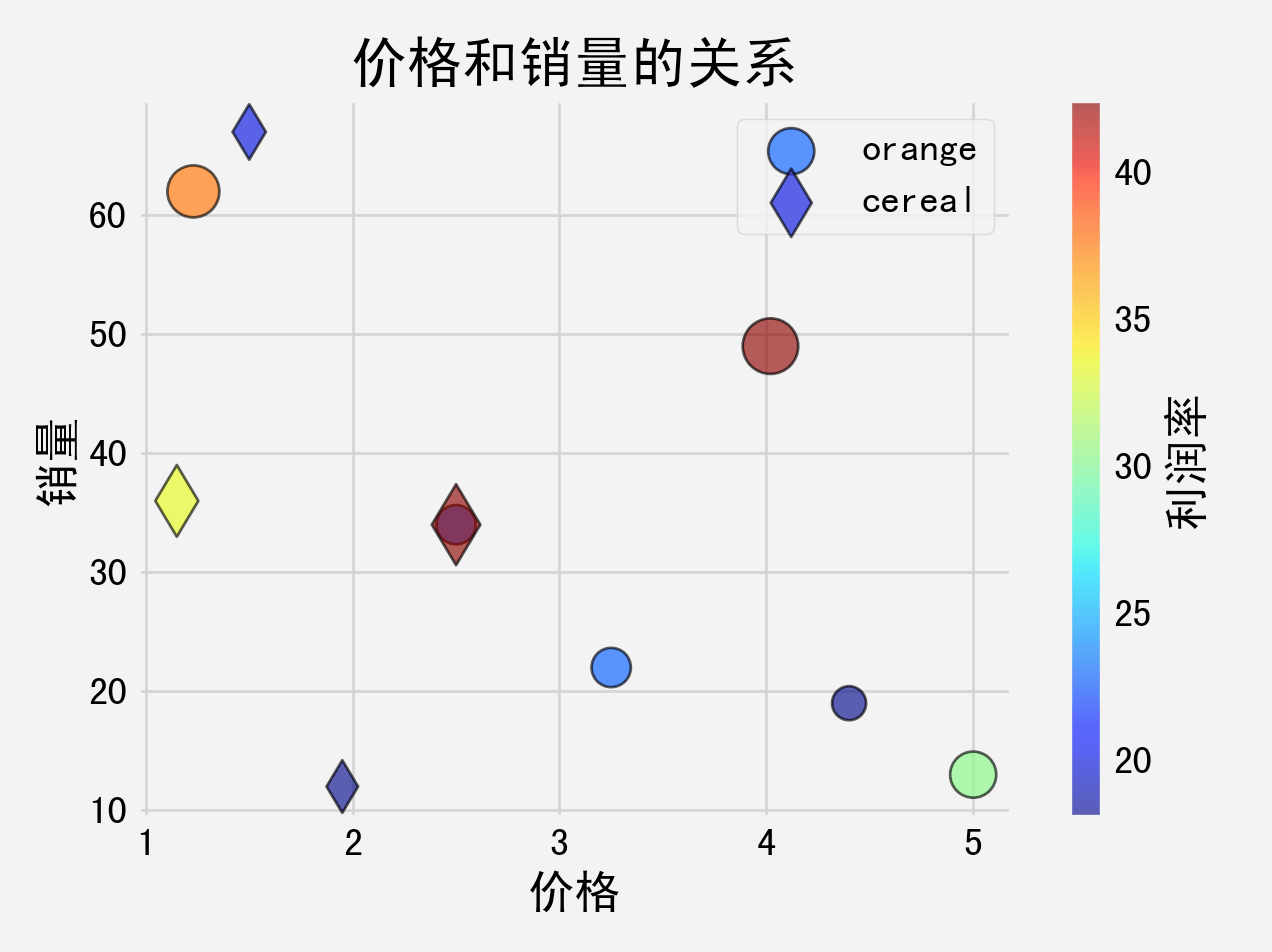• 系列文章目录
• 前言
• 一、什么是散点图？
• 二、散点图的绘制
• 三、应用场景
• 1.适用场景
• 2.不适用场景
• 总结

• # 前言

上一章我们讲述了直方图的绘制，本章我们来讲述散点图的绘制。

# 一、什么是散点图？

散点图，又名点图、散布图、X-Y图，英文 Scatter plot 或 Scatter gram。散点图将所有的数据以点的形式展现在平面直角坐标系上。它至少需要两个不同变量，一个沿 x 轴绘制，另一个沿 y 轴绘制。每个点在 x、y 轴上都有一个确定的位置。众多的散点叠加后，有助于展示数据集的“整体景观”，从而帮助我们分析两个变量之间的相关性，或找出趋势和规律。

散点图常被用于分析变量之间的相关性。如果两个变量的散点看上去都在一条直线附近波动，则称变量之间是线性相关的；如果所有点看上去都在某条曲线（非直线）附近波动，则称此相关为非线性相关的；如果所有点在图中没有显示任何关系，则称变量间是不相关的。

# 二、散点图的绘制

下面我们通过例子来讲述散点图的绘制，散点图的绘制使用 `scatter()` 函数。例如：

``````import matplotlib.pyplot as plt

plt.style.use('fivethirtyeight')

price = [2.50, 1.23, 4.02, 3.25, 5.00, 4.40]
sales_per_day = [34, 62, 49, 22, 13, 19]

plt.scatter(price, sales_per_day)

plt.title('价格和销量的关系')
plt.xlabel('价格')
plt.ylabel('销量')

plt.tight_layout()
plt.show()
``````

代码执行后得到的图形如下图所示：x 轴表示商品的价格，y 轴表示商品的销量，每个点表示一组数据。上面点的样式是默认的，我们可以通过设置参数的方式来改变点的样式，例如大小、颜色、形状等。

``````import matplotlib.pyplot as plt

plt.style.use('fivethirtyeight')

price = [2.50, 1.23, 4.02, 3.25, 5.00, 4.40]
sales_per_day = [34, 62, 49, 22, 13, 19]

plt.scatter(price, sales_per_day, s=100, c='green', marker='*')

plt.title('价格和销量的关系')
plt.xlabel('价格')
plt.ylabel('销量')

plt.tight_layout()
plt.show()
``````

上面的代码中，我们通过参数 s 设置了点的大小，c 设置了点的颜色，marker 设置了点的形状。执行完代码生成的图形如下图所示：同样地，我们还可以参数设置点的边缘颜色，点的透明度，例如：

``````import matplotlib.pyplot as plt

plt.style.use('fivethirtyeight')

price = [2.50, 1.23, 4.02, 3.25, 5.00, 4.40]
sales_per_day = [34, 62, 49, 22, 13, 19]

plt.scatter(price, sales_per_day, s=100, c='green', edgecolors='black', linewidth=1, alpha=0.7)

plt.title('价格和销量的关系')
plt.xlabel('价格')
plt.ylabel('销量')

plt.tight_layout()
plt.show()
``````

在上面的代码中，设置点的边缘的颜色为黑色，边缘线的粗细为 1，透明度设置成 0.7。执行完代码生成的图形如下图所示：上面的例子中，点的颜色是相同的，有时候我们需要根据另外一组数据来为点设置成不同的颜色，这样通过点的颜色便可以知道数据的大小。例如：

``````import matplotlib.pyplot as plt

plt.style.use('fivethirtyeight')

price = [2.50, 1.23, 4.02, 3.25, 5.00, 4.40]
sales_per_day = [34, 62, 49, 22, 13, 19]

profit_margin = [20, 35, 40, 20, 27.5, 15]

plt.scatter(price, sales_per_day, s=100, c=profit_margin, cmap='Greens', edgecolors='black', linewidth=1, alpha=0.7)

plt.title('价格和销量的关系')
plt.xlabel('价格')
plt.ylabel('销量')

plt.tight_layout()
plt.show()
``````

在上面的代码中，我们添加了一组代表利润率的数据 `profit_margin`，在绘制图形时，将参数 c 设置成 `profit_margin`，这样每个点都有一个自己的颜色值。代码执行后得到的图形如下图所示：上图中，颜色越深代表值越大，虽然从数据我们可以知道颜色越深代表值越大，但是为了让图形传达的信息更加精确，需要明确标识颜色和值的对应关系。例如：

``````import matplotlib.pyplot as plt

plt.style.use('fivethirtyeight')

price = [2.50, 1.23, 4.02, 3.25, 5.00, 4.40]
sales_per_day = [34, 62, 49, 22, 13, 19]

profit_margin = [20, 35, 40, 20, 27.5, 15]

plt.scatter(price, sales_per_day, s=100, c=profit_margin, cmap='Greens',
edgecolors='black', linewidth=1, alpha=0.7)

cbar = plt.colorbar()
cbar.set_label('利润率')

plt.title('价格和销量的关系')
plt.xlabel('价格')
plt.ylabel('销量')

plt.tight_layout()
plt.show()
``````

上面的代码中，通过 `colorbar()` 函数设置了色卡。代码执行后得到的图形如下图所示：上面是通过颜色的深浅来代表值的大小，在用颜色的深浅来代表值的大小的同时，我们还可以根据数据来设置点的大小。例如：

``````import matplotlib.pyplot as plt
import numpy as np

plt.style.use('fivethirtyeight')

price = np.array([2.50, 1.23, 4.02, 3.25, 5.00, 4.40])
sales_per_day = np.array([34, 62, 49, 22, 13, 19])

profit_margin = np.array([20, 35, 40, 20, 27.5, 15])

plt.scatter(price, sales_per_day, s=profit_margin*10, c=profit_margin, cmap='Greens',
edgecolors='black', linewidth=1, alpha=0.7)

cbar = plt.colorbar()
cbar.set_label('利润率')

plt.title('价格和销量的关系')
plt.xlabel('价格')
plt.ylabel('销量')

plt.tight_layout()
plt.show()
``````

上面的代码中，将点的大小设置成 profit_margin * 10，这样点的大小便和利润率成正比。代码执行后得到的图形如下图所示：上面的例子中只包含一组数据，在同一个图形中可以包含多组数据的散点图，例如：

``````import matplotlib.pyplot as plt
import numpy as np

plt.style.use('fivethirtyeight')

price_orange = np.array([2.50, 1.23, 4.02, 3.25, 5.00, 4.40])
sales_per_day_orange = np.array([34, 62, 49, 22, 13, 19])
profit_margin_orange = np.array([20, 35, 40, 20, 27.5, 15])

price_cereal = np.array([1.50, 2.50, 1.15, 1.95])
sales_per_day_cereal = np.array([67, 34, 36, 12])
profit_margin_cereal = np.array([20, 42.5, 33.3, 18])

plt.scatter(price_orange, sales_per_day_orange, s=profit_margin_orange*10, c=profit_margin_orange,
cmap='jet', edgecolors='black', linewidth=1, alpha=0.7, label='orange')

plt.scatter(price_cereal, sales_per_day_cereal, s=profit_margin_cereal*10, c=profit_margin_cereal,
cmap='jet', edgecolors='black', linewidth=1, alpha=0.7, marker='d', label='cereal')

cbar = plt.colorbar()
cbar.set_label('利润率')

plt.title('价格和销量的关系')
plt.xlabel('价格')
plt.ylabel('销量')

plt.legend()
plt.tight_layout()
plt.show()
``````

在上面的代码中，我们又添加了一组数据的点图，代码执行后得到的图形如下图所示：# 三、应用场景

## 1.适用场景

• 适用于分析变量之间是否存在某种关系或相关性。
• 适用于分析变量之间相关性的强弱，我们可以通过查看图上数据点的密度来确定相关性的强弱。
• 适用于在不考虑时间的情况下比较大量的数据点，数据点越多，比较的效果就越明显。
• ## 2.不适用场景

• 对于数据量较少的数据集不建议使用，分析结果会存在较大的偶然性。
• 不适用于数据点过大、过多的情况，会影响图表的可读性，导致无法进行分析。可以通过减小点的大小、调整透明度、减少数据量、数据分组、建立3D模型等等的方法进行优化。
• 数据分类过多，无法快速识别，失去可视化的意义和价值。
• 通过观察散点图得出的变量之间的相关性并不等同于确定的因果关系。

• # 总结

本章我们讲述了散点图的绘制以及散点图的适用场景和不适用场景。

来源：mr_songw